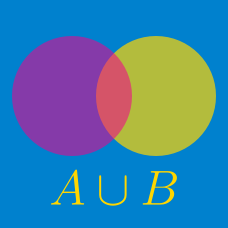Probability

# Sets - Symmetric Difference

Consider the sets \begin{align} A &=\{x \mid -8 < x < 4, x \text{ is an integer} \}\\ B &=\{x \mid -2 < x \leq 11, x \text{ is an integer} \}. \end{align} What is $$\lvert A \triangle B \rvert ?$$

Details and assumptions

You may choose to read the summary page Set Notation.

Consider two sets:

$A = \{ 5, 6, 7, 8, 9, 10 \} \\ B = \{ 6, 8, 10, 16, 24 \}$

What is $$\lvert A \triangle B \rvert$$?

Consider the sets $A=\{1,2,a+7\}, B=\{a+3, a^2, -a+10, 16\}.$ If $$A \cap B=\{1, 16\},$$ what is the sum of all the elements in the set $$A \triangle B?$$

Details and assumptions

You may choose to read the summary page Set Notation.

If sets $$A$$ and $$B$$ satisfy \begin{align} A & =\{23, 11, 55, 6, 4, 18, 37 \},\\ A\cap B & =\{37, 55\}, \\ \lvert A \triangle B \rvert & =5, \end{align} what is the sum of all the elements in the set $$B?$$

Details and assumptions

You may choose to read the summary page Set Notation.

If $$A$$ and $$B$$ are two sets such that $|A|=24, |B|=17, |B-A|=9,$ what is the value of $$\lvert A \triangle B \rvert ?$$

Details and assumptions

You may choose to read the summary page Set Notation.

×